aras

•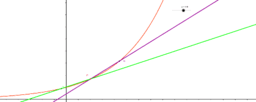the secant becomes a tangent

Activity

aras

•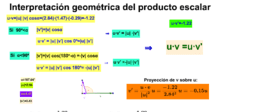Interpretación geométrica del producto escalar

Activity

aras

•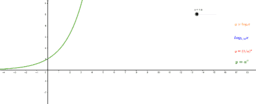one chart, four graphs

Activity

aras

•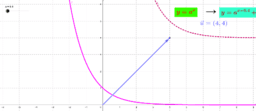exponential and logarithm functions

Activity

aras

•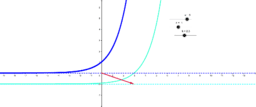translating exponentials

Activity

aras

•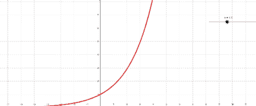exponential functions

Activity

aras

•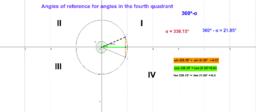Reference angles for the fourth quadrant

Activity

aras

•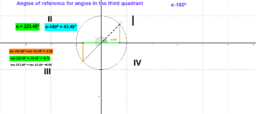Activity

aras

•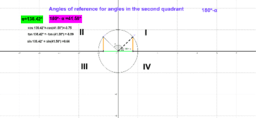Angles of reference for second quadrant angles

Activity

aras

•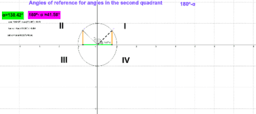Activity

aras

•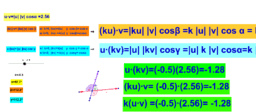Activity

aras

•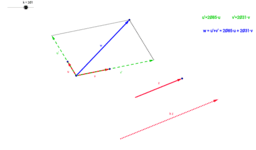vectors as linear combinations of a base

Activity

aras

•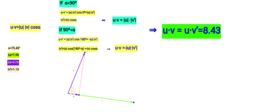Dot Product. Geometrical view

Activity

aras

•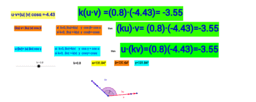Homogeneity of the dot product

Activity

aras

•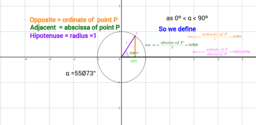Trigonometric ratios

Activity

aras

•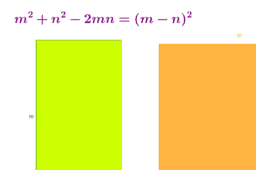The square of a difference

Activity

aras

•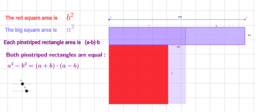Difference of two squares

Activity

aras

•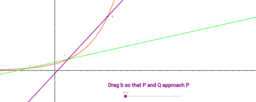limit of secant lines

Activity

aras

•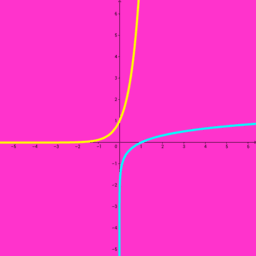Activity

aras